This post will teach you how to quickly generate a random password with Formula in Excel. You can combine a series of worksheet functions to create a complex formula to generate a random password in Microsoft Excel.

Normally, a secure password should have 8 characters at least and contain a mix of uppercase letters, lowercase letters, numbers and one specific character. To generate a Random and secure password in Excel, you can use a combination of the CHAR function, the RANDBETWEEN function and concatenation operator to build a new Excel formula. Just refer to the following formula.

`=CHAR(RANDBETWEEN(65,90))&CHAR(RANDBETWEEN(97,122))&CHAR(RANDBETWEEN(97,122))&CHAR(RANDBETWEEN(65,90))&RANDBETWEEN(100,999)&CHAR(RANDBETWEEN(42,43))`

Select one cell that you want to generate the random password, then enter this formula in that Cell, and press Enter key to apply this formula. You will see that it will generate an eight characters password.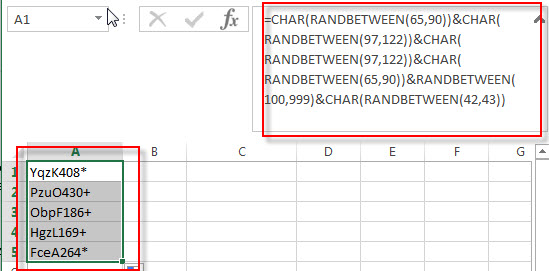If you want to generate a random password only contain alpha characters (random uppercase letter string), you can use the following formula:

`=CHAR(RANDBETWEEN(65,90))`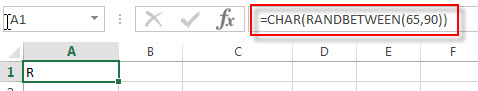If you want only to generate a random lowercase letter string, you can use the below formula:

`=CHAR(RANDBETWEEN(97,122))`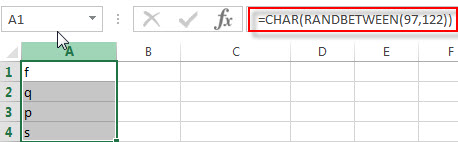If you want to generate a random password only contain 6-digit numeric characters, you can use the following formula:

`=RANDBETWEEN(100000,900000)`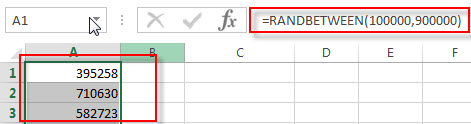If you want to generate a random symbol string, just use the following formula:

`=CHAR(RANDBETWEEN(33,47))`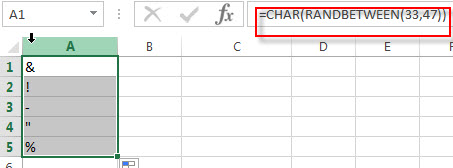This formula will randomly generate one of the following specific characters: !”#\$%&'()*+,-./

We talked that the CHAR function will generate lots of specific characters, and if you just want to create a password that can be type on the keyboard, so you should keep the specific character simple and avoid to use characters like ¶, Œ, or ©.

### Related Functions

• Excel CHAR function
The Excel CHAR function returns the character specified by a number (ASCII Value).The CHAR function is a build-in function in Microsoft Excel and it is categorized as a Text Function. The syntax of the CHAR function is as below:=CHAR(number)….
• Excel RANDBETWEEN Function
The Excel RANDBETWEEN function returns a random integer number that is between the numbers you specify.The syntax of the RANDBETWEEN function is as below:= RANDBETWEEN (bottom,top)….

Related Posts

How to Generate Random Values by a List in Different Cases in Excel

Sometimes we may create some fake data for testing or some other purpose. If we want to create an amount of numbers, we may feel it is annoying by entering random numbers one by one. For this instance, we can ...

How to Pick a Random Name from a List in Excel

This post will guide you how to pick a random name from a list in Excel. How do I select random value from a list or a table with a formula in Excel 2013/2016. Pick Random Name from a List ...

How to Generate Random Numbers between Two Numbers in Excel

This post will guide you how to generate random numbers between two numbers in Excel. How do I generate random numbers between two numbers without duplicates with VBA macro in Excel. Generate Random Numbers between Two Numbers with Formula Generate ...

Randomly Select Cells

This post will guide you how to randomly select cells from a list or table in Excel. How to get random value form a range of cells in an Excel Spreadsheet. Assuming that you have a list of data (B1:B7) ...

Generate Random Character Strings

This post will guide you how to generate a random character string in excel. How do I create a random passwords, text strings with a formula in excel. How do I generate random strings of characters with VBA code in excel. ...

Excel XIRR Function

This post will guide you how to use Excel XIRR function with syntax and examples in Microsoft excel. Description The Excel XIRR function returns the internal rate of return for a series of cash flows that is not necessarily periodic. ...

Excel VDB Function

This post will guide you how to use Excel VDB function with syntax and examples in Microsoft excel. Description The Excel VDB function calculates the depreciation of an asset for a specified period based on the double declining balance method. ...

Excel SYD Function

This post will guide you how to use Excel SYD function with syntax and examples in Microsoft excel. Description The Excel SYD function calculates the sum-of-years’digits depreciation of an asset for a specified period. And it will return a numeric ...

Excel SLN Function

This post will guide you how to use Excel SLN function with syntax and examples in Microsoft excel. Description The Excel SLN function calculates the depreciation of an asset for one period based on the straight line depreciation. And it ...

Excel PV Function

This post will guide you how to use Excel PV function with syntax and examples in Microsoft excel. Description The Excel PV function returns the present value of a loan or investment based on constant payments and a constant interest ...

Sidebar